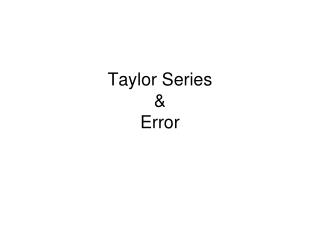DownloadDownload PresentationTaylor Series & Error

# Taylor Series & Error

Télécharger la présentation## Taylor Series & Error

- - - - - - - - - - - - - - - - - - - - - - - - - - - E N D - - - - - - - - - - - - - - - - - - - - - - - - - - -
##### Presentation Transcript

1. Taylor Series&Error

2. Series and Iterative methods Any series ∑xncan be turned into an iterative method by considering the sequence of partial sums sn. Functions and Taylor Expansions From Intermediate Calculus we know any function that has n+1 derivatives at a point a has a nth Taylor Polynomial expansion centered at a and an expression for the error. The value f(k)(a) is the kth derivative evaluated at a. The function Rn(x) represents the error where c is a value between x and a.

3. Examples of Taylor Series Because the higher derivatives of some functions repeat in a recognizable pattern we are able to get a few "standard" Taylor Series expansions. All of the examples below have the center point of 0 and the error term will go to zero for suitable values of x. Other more complicated functions can be found using the algebra, derivatives and integrals.

4. To find the Taylor series for the function f(x) = arctan(x). Start with known Taylor Series Substitute –x for x Substitute x2 for x Integrate both sides. To find the Taylor series for the function Start with known Taylor Series Substitute Multiply by x3

5. A second version of Taylor Polynomials For any function f(x) consider the function f(x+h) where we think of x as a constant and h is the variable. Consider the Taylor expansion for f(x+h) (with variable h) centered at 0. The kth derivatives are found to the right. This is a more computationally useful way of applying Taylor Series to approximate functions. We think of the value of h as small (close to zero) and will represent the distance between computable derivatives and x. Consider the following example. Approximate sin(30.01º) with an error of less than 0.00000001. We first need to convert to radians to get the value for h.

6. We need to figure out the value of k that gives the desired accuracy. The exact error is given by the expression to the right. We know the values for sine and cosine are always less than 1 in absolute value and  is less than 4. Try different values of k that make this inequality true. When we try k=2 we find the right side is 0.000000000001829. This means we need go out to the k=2 term.

7. Alternating Series and Error From intermediate calc remember that a series ∑(-1)kak converges if the sequence ak are positive terms that decrease to zero, this was called the alternating series test. The series ∑(-1)kak is called an Alternating Series. The nice thing about alternating series is that keeping tract of the error is very easy as given by the theorem below. Alternating Series Error Theorem In any convergent alternating series ∑(-1)kakthe error is always less than the first term omitted. In other words: |Error| < ak+1 Example: Compute 1/e accurate to .0001 The series for 1/e is alternating so the theorem applies. We need to go to the k=7 term.